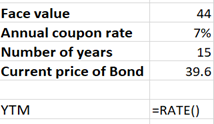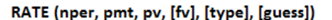# Olympic Sports has two issues of debt outstanding. One is a 6% coupon bond with a face value of \$39 million, a maturity of 10 years, and a yield to maturity of 7%. The coupons are paid annually. The other bond issue has a maturity of 15 years, with coupons also paid annually, and a coupon rate of 7%. The face value of the issue is \$44 million, and the issue sells for 90% of par value. The firm's tax rate is 35%. a. What is the before-tax cost of debt for Olympic?b. What is Olympic's after-tax cost of debt?

Question
2 views

Olympic Sports has two issues of debt outstanding. One is a 6% coupon bond with a face value of \$39 million, a maturity of 10 years, and a yield to maturity of 7%. The coupons are paid annually. The other bond issue has a maturity of 15 years, with coupons also paid annually, and a coupon rate of 7%. The face value of the issue is \$44 million, and the issue sells for 90% of par value. The firm's tax rate is 35%.

a. What is the before-tax cost of debt for Olympic?

b. What is Olympic's after-tax cost of debt?

check_circle

Step 1

Given,

Bond one: Coupon rate =6%, face value =\$39 million, maturity period =10 years, YTM=7%, paid annually.

Another Bond : Coupon rate =7%, face value =\$44 million, maturity period =15 years, YTM=? current price of bond = 39.6 (selling at 90% of face value), paid annually.

Required to calculate: before and the after-tax cost of debt for Olympic.

YTM is considered as r = cost of debt, for bond one YTM is already given so here we need to calculate YTM=r for another bond.

Step 2

r=YTM= cost of debt can be calculated manually as well as using the RATE function of Excel, here we are using Excel:

Put the following values while using rate function:Put the following values while using rate function,

Nper = total number of payment periods =15

Pmt = payment for each period =44*7% =3.08

Pv = current price of bond, always write it in =-39.6

FV = Value at maturity here it is face value =44

Type = 0

Guess = does not require

=RATE(15,3.08,-39.6,44,0)

=8.18%

YTM= 8.18% after rounding off it will be 8%

Step 3

a)Now pre-tax cost of debt for Olympic can be calculated follows:

pre-tax cost of debt = cost of debt for bond one + cost of debt for bond two

&n...

### Want to see the full answer?

See Solution

#### Want to see this answer and more?

Solutions are written by subject experts who are available 24/7. Questions are typically answered within 1 hour.*

See Solution
*Response times may vary by subject and question.
Tagged in